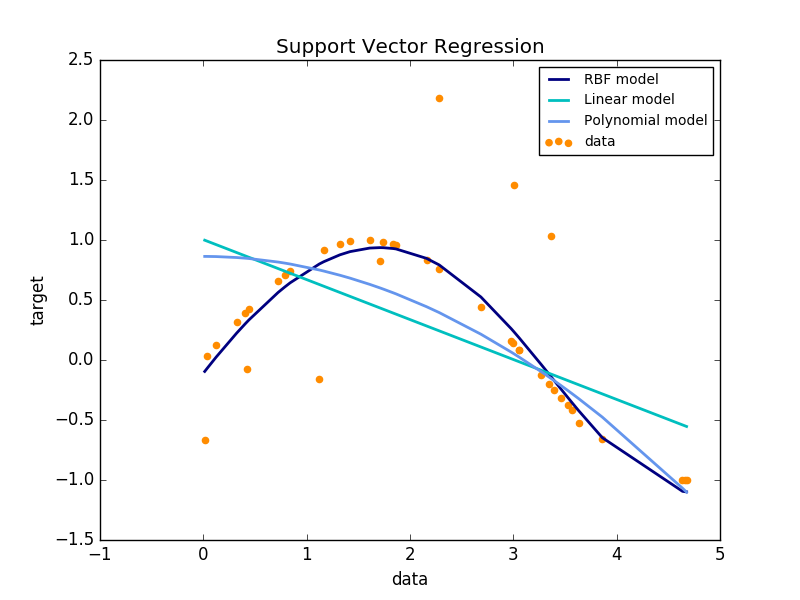# REGRESSION FOR BUSINESS, USING R & PYTHON: PART I – INTRO

Every Business should know varied factors, which influence their Operation and Revenues. Identifying these key elements will eventually, lead to improving the design of the business structure but how to identify the significant factors? Moreover, how to predict the outcome caused by these factors? Regression can be the key to all these questions. Let us see how regression can be brought into play for a business.

### APPLICATION OF REGRESSION IN BUSINESS

For these applications firstly, Data generated by the business is needed follwed by Regression Model, which perfectly fits the data.

#### PREDICTIVE ANALYTICS:

Predictive Analytics means forecasting the requirements of a business like revenue of a firm or demand in the market. It can be used to increase the productivity and operation efficiency or to reduce the cost and inventory levels etc.

Example: Predictive analytics can help forecast the customers who have the highest probability of buying your product, then send the coupon to only those people to optimize the revenue.

#### SUPPORTING DECISIONS:

Regression analysis can bring a scientific value to the decisions made in businesses, which can eliminate intuition. Data from various departments of a business is used to run a regression model thereby arriving at a conclusion on business decisions.

Example: A manager can decide whether to expand his production by estimating the sales.

#### CORRECTING ERRORS:

A regression analysis can identify the errors in the decisions made and used to rectify the errors.

Example: a business wants to improve sales by 20% by reducing the price by 10% but regression analysis may predict only 5% increase in sales. Thereby correcting the decision.

#### OPERATION EFFICIENCY:

We can optimize the business operations by predicting the demand, resource, workforce etc.

Example: optimum inventory can be estimated for maximizing the production.

#### NEW INSIGHTS:

By analyzing the data of a business, new dimensions in that business can be unlocked which will help in the expansion of the business.

Example: By using regression analysis new potential segments in the market can be found.

### SO, WHAT IS REGRESSION?

Regression, in layman terms, is a Cause and Effect relationship in which, an Independent Variables would be varied and controlled in an experiment in order to test their effects on the Dependent Variable.

The overall idea of regression is to examine two things i.e. Does a set of Independent variables predict an outcome (dependent) variable? Moreover, which variables in specific are significant predictors of the dependent variable and in which way do the magnitude and sign of Independent variable impact the dependent variable.

Following equation represents the general regression function

# Y = f(X1, X2 ….Xn)

Y is Dependent Variable, X1, X2 ….Xn are Independent Variables

Let us look at Aggregate Demand of a country, which is dependent on various factors like Consumer Spending, Investment (Gross Fixed Capital Formation), Government Spending, Exports, Imports.

# AD = C + I + G + X – M

C is Consumer spending

‘I’ is Investment (Gross Fixed Capital Formation)

G is Government Spending

X is Exports

M is Imports

Here AD is dependent variable whereas C, I, G, X, M are independent variables. With this regression model, Government can know how to affect the economy of the country by changing any independent variable.

### COEFFICIENT OF REGRESSION

Coefficients are the values which cause multiplier change to the dependent variable with respect to the independent variable. The magnitude and direction (sign) of a coefficient in an equation affect the dependent variable. These coefficients have multiplier effect i.e. for example, if X (independent variable) has a coefficient of positive two then, for every one unit increase in X results in two units rise in Y(Dependent Variable).

# Y = A+B1X1+ B2X2+…+ BnXn

Here B1, B2, and Bn are coefficients of X1, X2, and Xn respectively.

### TYPES OF REGRESSION

Regression is more complex as we dig deep into it. Every data need to be analyzed by fitting different regression models for best predictions. There are innumerable regression models, which can be used for analysis. Each model has its own importance and specific conditions.As shown above, not all regression models can be the best fit for every dataset. So depending on the nature of data, models are selected for fitting.

FEW TYPES OF REGRESSION MODEL

• Linear
• Logistic
• Ridge
• Bayesian
• Ecologic
• Lasso
• SVR
• Decision Trees
• Random Forest
• Polynomial etc.

I will explain in-depth about few important regression models and machine learning in my upcoming articles.

### MACHINE LEARNING: REGRESSION

When data of a business is voluminous and complex that traditional data processing application software is inadequate to deal with. This data is known as Big Data. We need statistical computing software like R Language and Python to process and analyze the data. Ergo Machine Learning models are used for the analysis.

In coming up articles, I would be explaining about Different regression models by using machine learning in R and Python. Stay tuned. I would like to thank my friend, Miss Lavanya D. for her support. Thank you for reading.

Avinash Reddy